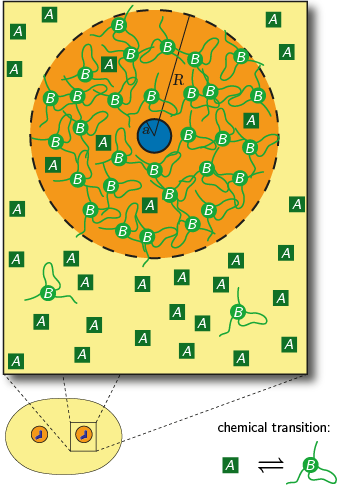## Physical description of centrosomes

We describe centrosomes as protein droplets that are assembled from building blocks. This assembly process is controlled by chemical reactions driven by enzymes. Consequently, we speak of active droplets.Figure 1: Schematic drawing of the cell, where pairs of centrioles (blue) are surrounded by PCM (orange) and immersed in the cytosol (yellow). The magnification on the top shows soluble building blocks of form A (dark green squares) both in the cytosol and in the centrosome. Chemical reactions turn form A into the droplet material B (green discs) that forms a gel defining the PCM.

We describe the centrosome as a liquid-like droplet of pericentriolar material (PCM) that forms around a pair of centrioles. We propose that the centrosome material exists in a soluble and in a phase separating form. Chemical reactions controlling the transitions between these forms account for the temporal evolution observed in experiments. Interestingly, the destabilizing effect of surface tension can be counteracted by an enzymatic activity of the centrioles, which are present at the core of all centrosomes. This non-equilibrium feature also allows for multiple stable structures, a situation which is unstable in equilibrium phase separation. Our example establishes a general picture of the organization of membrane-less organelles.

The simulations presented here capture these physics in a simple model, see Fig. 1. We consider a closed system of volume Vc with an average fraction ϕ of PCM components, such that its total amount is ϕVc. The system contains N centriole pairs, which we each approximate by spheres of radius a. The model describes the dynamics of PCM accumulation around these stable cores. Here, we distinguish the soluble form A and the phase separation form B of the PCM components. Only the latter form droplets around the centrioles. The chemical transitions between these two forms are described by several different chemical reactions: first-order reactions A ⇌ B quantified by the rate constants kAB and kBA, an autocatalytic reaction A + B → 2B with rate constant kc, which happens predominately inside the droplet, and a catalytic activity Q at the surface of the centriole pair.

The droplets form because the phase separating form B segregates from the cytosol. We assume that the volume fraction ψ- of form B inside the droplet is fixed. If the interface between the droplet and the cytosol is in thermodynamic equilibrium, there will also be some droplet material right outside the droplet. We approximate its volume fraction by ɣβ+/R, where ɣ/R is the Laplace pressure caused by the surface tension ɣ of the curved interface of the spherical droplet of radius R. Here, β+ is a suitable proportionality constant that determines the strength of the influence of surface tension. The droplet material outside of the droplet is subjected to the chemical reactions introduced above. These chemical reactions lead to compositional gradients, which then cause material fluxes due to the diffusivity DB of the droplet material. For simplicity, we assume that the soluble building blocks of form A diffuse fast in the model presented here.